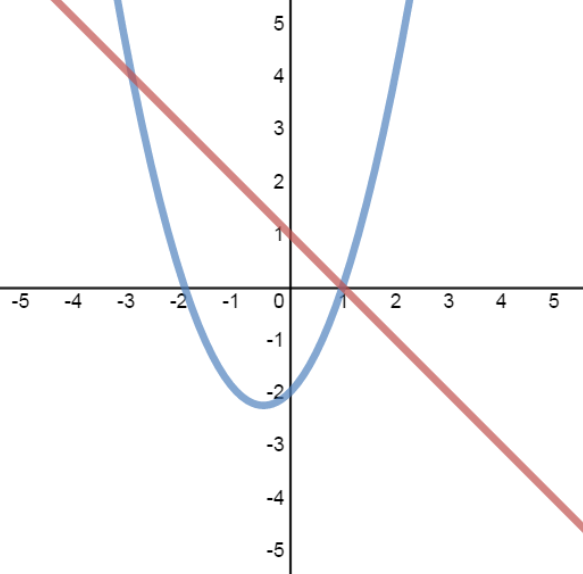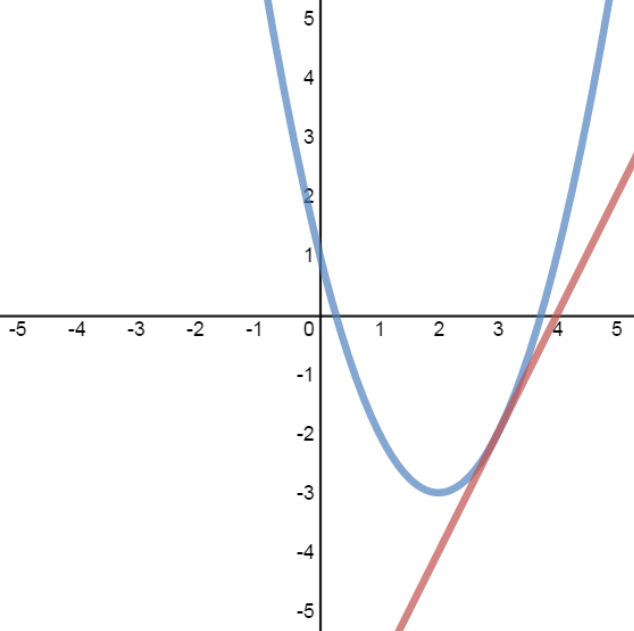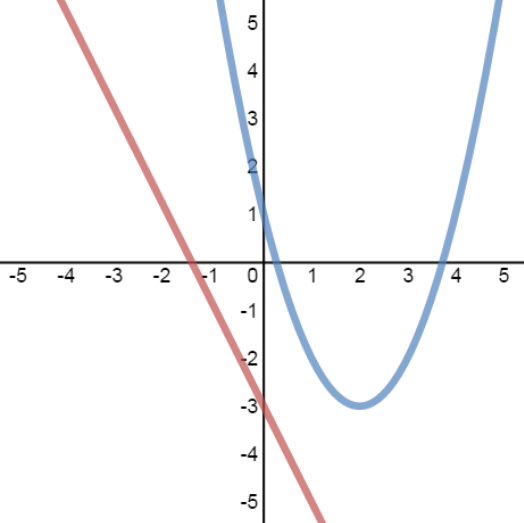The solutions to a system of equations are the points of intersection of their graphs. There are 3 cases you will come across when trying to solve the system. There can be 2 solutions, 1 solution or even no solutions.

#### Lessons

• Introduction
• The solutions to a system of equations are the points of intersection of the graphs.
• For a system consisting of a linear equation and a quadratic equation:
linear equation: $y = mx + b$
quadratic equation: $y = a{x^2} + bx + c$
There are 3 cases to consider:

case 1: 2 solutions case 2: 1 solution case 3: no solutions• 1.
Case 1: System with 2 Solutions
a)
Solve the system:
$y = - x + 1$
$y = {x^2} + x - 2$

b)
Verify the solutions graphically

• 2.
Case 2: System with 1 Solution
a)
Solve the system:
$2x - y = 8$
$y = {x^2} - 4x + 1$

b)
Verify the solutions graphically

• 3.
Case 3: System with No Solutions
a)
Solve the system:
$10x + 5y + 15 = 0$
$y = {x^2} - 4x + 2$

b)
Verify the solutions graphically